# Peirce's Rules of Inference

John F. Sowa

#### Abstract

The rules of inference for conceptual graphs are based on Peirce's rules of inference for existential graphs. Those rules, which are the simplest and most elegant of any rules every proposed for first-order logic, are general enough to derive all other rules of inference as special cases. This paper starts with a summary of Peirce's original rules of inference, presents their extension to conceptual graphs, and generalizes them as universal rules of inference that can be applied to logic in any notation whatever. In particular, it shows that both Gentzen's rules of natural deduction and Robinson's rules of resolution are special cases of Peirce's rules. When suitably generalized, Peirce's rules can be applied to any notation for logic, including the common Peirce-Peano notation for predicate calculus or even a version of controlled natural language.

Readers who would like to read Peirce's own tutorial on existential graphs should see the annotated version of his Manuscript 514. For more background on Peirce's role in the history of logic, see the articles by Putnam (1982), Quine (1995), Dipert (1995), and Hintikka (1997).

## 1 Peirce's Logic from Algebra to Graphs

Peirce's algebraic notation for logic, with a change of symbols Peano, became the most widely used notation for logic during the 20th century. Peirce developed that notation as a generalization of Boolean algebra (Boole 1847, 1854), which he extended in a variety of ways during the 1870s. Following are some of his many innovations:

• The extension of Boolean algebra to relational algebra for representing combinations of monadic and dyadic relations.

• The claw symbol, p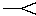q, for material implication, which indicates that the truth value of p is less than or equal to the truth value of q (with the representation of truth by 1 and falsity by 0).

• The interpretation of disjunction, p + q, as inclusive-or instead of Boole's interpretation as exclusive-or.

• The use of truth tables as a general method for determining the validity or satisfiability of any proposition in Boolean algebra.

• The discovery that any Boolean proposition can be stated in terms of a single dyadic operator, representing not-both or not-either.
During the 1880s, while he was editing the second edition of his father's book on linear algebra, Peirce began to use subscripts to indicate the individuals that were related by any relation. With the use of subscripts, Peirce could represent relations with any number of arguments (or as he called them, subjects). He also adopted the symbols Σ and Π from linear algebra to indicate repeated logical sums (disjunctions) and logical products (conjunctions). The following statement, for example, would show that the monadic predicate P is true for at least one individual i:
ΣiPi > 0.
If P is false for every value of i, then the repeated disjunction would be 0. But if P is true for at least one i, then the repeated disjunction would be 1. By a similar argument, the repeated conjunction would be greater than 0 only if P is true for every individual i:
ΠiPi > 0.
In 1880, Peirce used this notation to prove various theorems that are now known to be true for the existential and universal quantifiers. Peirce, however, graciously attributed the invention of the quantifier to one of his students, who observed that the appendage ">0" was superfluous. In 1885, Peirce used this notation in his publication of the complete rules of inference for what he called first intentional and second intentional logic. In that paper, Peirce coined the terms existential quantifier for Σ and universal quantifier for Π. Schröder translated intentional in the German Ordnung, and Russell translated the terms back into English as first-order and second-order logic. As early as 1869, Peirce gave model-theoretic arguments to demonstrate the soundness of his extensions to the rules of inference of Boolean algebra:
All that the formal logician has to say is, that if facts capable of expression in such and such forms of words are true, another fact whose expression is related in a certain way to the expression of these others is also true.... The proposition 'If A, then B' may conveniently be regarded as equivalent to 'Every case of the truth of A is a case of the truth of B.'
Peirce used such arguments to justify his rules of 1885, but he did not formalize his model theory in as much detail as Tarski (1933, 1936).

Although Frege (1879) had published the first complete system of first-order logic, no one else ever adopted his notation. Ernst Schröder (1895) adopted Peirce's notation for his three-volume Vorlesungen über die Algebra der Logik, which became the most widely used textbook on logic until Whitehead and Russell published the Principia Mathematica in 1910. Giuseppe Peano (1889) adopted the notation from Peirce, but he changed the symbols because he wanted to mix mathematical and logical symbols in the same formulas. Peano was aware of Frege's work and published a negative review, which declared Frege's notation "unreadable." That review led to a correspondence between Frege and Peano, but Peano refused to read Frege's discussion unless he translated his diagrams to the Peirce-Peano notation. In Germany and Poland, the early work on logic by Hilbert, Zermelo, Löwenheim, Tarski, and others was done in Peirce's notation. In 1931, Gödel still used Π for the universal quantifier. In Polish notation, Peirce's symbols Σ and Π are still used for the quantifiers.

In England, Whitehead (1898) referred to Peirce's work of the 1870s and 1880s in his book Universal Algebra. But Russell, who was never known for intellectual generosity (or even honesty), said that he first learned the notation from Peano and reinvented everything himself before he came across Frege's work. In the preface to the Principia Mathematica, Russell credited Frege and Peano, but he did not mention Peirce or Schröder. Under Russell's influence, the Peirce-Peano notation came to be called Peano-Russell notation, despite the fact that Russell hadn't added or modified anything in it.

Although Peirce began to experiment with graph notations for logic in 1882, he didn't discover a notation that was as complete as his algebraic notation until 1896, with his entiatitive graphs, which were based on graphical symbols for negation, disjuction, and the universal quantifier. The following year, he switched to the dual form existential graphs, with symbols for negation, conjunction, and the existential quantifier. For the remainder of his life, Peirce used existential graphs for most of his work on logic.

Peirce discovered the rules of inference for existential graphs in 1897, and he restated them with minor variations in various publications and manuscripts. The version presented here is based on his Manuscript 514, which he wrote in 1909. To illustrate the rules, Figure 1 shows two of Peirce's existential graphs from MS 514. The graph on the left may be read "There is something of thunder and not lightening." The graph on the right may be read "If there is something of thunder, then it is lightening."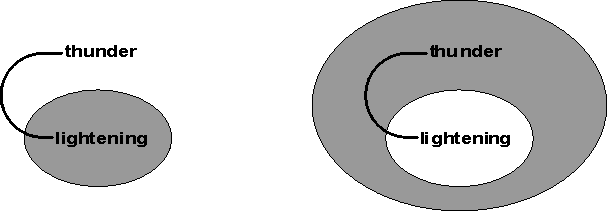Figure 1:  Two existential graphs

In an existential graph, a bar, which Peirce called a line of identity, represents something; it corresponds to an existentially quantified variable in the algebraic notation. The curved bar in the graph on the left, for example, represents something, which is linked to a monadic predicate named thunder. The combination therefore asserts that there exists something which is thunder. A shaded oval represents the negation of any graph or subgraph contained in it. Therefore, the part of the graph which is negated by the shaded oval asserts that the certain something is not lightening. Altogether, the graph on the left may be translated to the following formula in Peirce-Peano notation:

(∃x)(thunder(x) ∧ ~lightening(x)).
This formula says that there exists something x, which is thunder and not lightening. The graph on the right of Figure 1 contains a nest of two ovals. The outer oval, which is shaded, represents a negation; the inner oval, which is unshaded, represents the negation of a negation. Altogether, the combination asserts "It is false that there exists something which is thunder and not lightening." In the algebraic notation, that sentence could be represented by the following formula:
~(∃x)(thunder(x) ∧ ~lightening(x)).
This formula says that it is false that there exists something x, which thunders and not lightens. By Peirce's 1885 rules for quantifiers, the negation at the front of the formula can be moved after the existential quantifier provided that the existential quantifier is converted to a universal quantifier. Then the combination ~(p ∧ ~q) can be converted to an implication. In Peirce-Peano notation, the entire formula would be
(∀x)(thunder(x) ⊃ lightening(x)).
This formula says that for every x, if x thunders, then x lightens. Peirce, however, preferred to treat a nest of an unshaded oval inside a shaded oval as a single implication, which he read "If it thunders, it lightens."

After this brief survey of Peirce's notation, it is possible to state Peirce's rules of inference. Readers who would like more examples and discussion about existential graphs should read his tutorial in MS 514. Peirce called his inference rules permissions because they state the permissible transformations of existential graphs that preserve truth — they never convert a true statement to a false statement (although the rules might sometimes convert a false statement to a true statement). Peirce's only axiom is the empty graph, which asserts nothing and is never false. Peirce's axiom, in fact, is the same as Gentzen's axiom for his version of natural deduction.

In MS 514, Peirce stated his rules as three permissions; each of which could be considered as a pair of rules. The same rules apply to propositional logic and to predicate logic. Since EGs have no variables, the rules for dealing with variables in the algebraic notation are replaced by simpler rules for cutting or connecting lines of identity.

Peirce's first permission consists of one rule that allows erasures in an unshaded area (either not nested at all or nested inside an even number of negations) and another rule that allows insertions in a shaded area (nested inside an odd number of negations). What is erased or inserted may be an entire graph or just a part of a graph, such as part of a line of identity.

1st Permission. Any graph instance on an unshaded area may be erased; and on a shaded area that already exists, any graph instance may be inserted. This includes the right to cut any line of identity on an unshaded area, and to prolong one or join two on a shaded area. (The shading itself must not be erased of course, because it is not a graph instance.)
To prove that his rules are sound, Peirce invented a version of model theory, which he called endoporeutic or outside-in evaluation. To show that these rules preserve truth, he observed that erasing graphs in an unshaded (positive) area reduces the number of conditions that might be false. Therefore, a false area might become true, but a true area cannot become false. The act of inserting graphs in a shaded (negative) area increases the number of conditions that might be false. Therefore, a true area might become false, but a false area cannot become true; the negation of that shaded area might switch from false to true, but it cannot switch from true to false.

The operations on lines of identity have the similar effects of erasing conditions in a positive area or adding conditions in a negative area. Cutting a line in an unshaded (positive) area eliminates a condition (the equality of two individuals) that might be false. By erasing the equality, cutting a line allows either end to be assigned independently to different individuals in a model.

Connecting two lines in a shaded (negative) area introduces a condition that might be false. That option is equivalent to universal instantiation, because an existential quantifier in a negative area has the effect of a universal quantifier. The result of connecting a line that represents something x to a line that represents a universal quantifier (∀y) has the effect of replacing y by x.

The second permision consists of two rules, called iteration and deiteration. Iteration copies graphs or subgraphs from an outer context to a more deeply nested context. Deiteration is the inverse, which erases a copy that might have been created by iteration (whether or not it was actually so created). Neither of these two rules has any effect on the truth value of a graph.

2nd Permission. Any graph-instance may be iterated (i.e. duplicated) in the same area or in any area enclosed within that, provided the new lines of identity so introduced have identically the same connexions they had before the iteration. And if any graph instance is already duplicated in the same area or in two areas one of which is included (whether immediately or not) within the other, their connexions being identical, then the inner of the instances (or either of them if they are in the same area) may be erased. This is called the Rule of Iteration and Deiteration.
In other writings, Peirce explained the implications of maintaining "identically the same connections" of the lines of identity: iteration extends a line from the outside inward, and deiteration retracts a line from the inside outward. By iteration, any line of identity may be extended in the same area or into any enclosed area. By deiteration, any end of a line of identity that is not attached to another line or to some relation name may be erased, starting from the innermost area in which it occurs.

By endoporeutic, Peirce showed that the rules of iteration and deiteration can never change the truth value of a graph. Since the evaluation of the truth value of a graph is calculated from the outside inward (which is his definition of endoporeutic), the value of each graph or subgraph is determined at the point when the method of evaluation reaches it. If a subgraph g has the value true at any point, no copies of g can affect the truth value of the current area or any enclosed area. If g has the value false, the current area must already be false, and no copies of g in the current area or any enclosed area can make the current area true.

A double negation is a ring-shaped area (shaded or unshaded) that contains nothing except possibly for lines of identity that originate outside the outer oval and continue into the inner oval without making any connections to anything in between. The third permission says that any double negation may be erased or inserted around any set of graphs. Following is Peirce's statement:

3rd Permission. Any ring-shaped area which is entirely vacant may be suppressed by extending the areas within and without it so that they form one. And a vacant ring shaped area may be created in any area by shading or by obliterating shading so as to separate two parts of any area by the new ring shaped area.

Erasing or inserting a double negation can have no effect on the truth value of a graph because not-not-true is true, and not-not-false is false. Therefore, truth is preserved.

As an application of these rules, it is possible to derive a contradiction from the two graphs in Figure 1. The first step is to apply the rule of iteration (second permission) to extend the line of identity from the graph on the left into the shaded area of the graph on the right. Then by the rule of insertion (first permission) the extended line may be joined to the line of identity already in the shaded area. The result is Figure 2.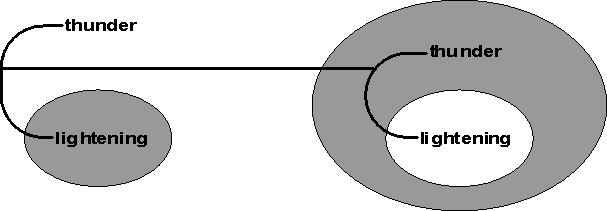Figure 2:  Result of iteration applied to Figure 1

Now the subgraph "—thunder" in the graph on the right is connected to the same line of identity as the subgraph "—thunder" in the outer area. By the rule of deiteration (second permission), the inner copy may be erased to produce Figure 3.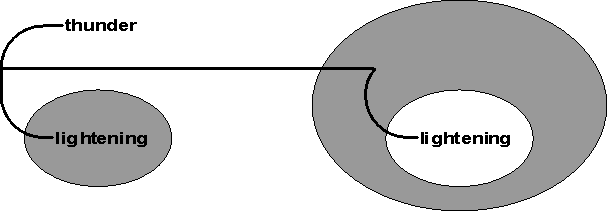Figure 3:  Result of deiteration applied to Figure 2

Now there is a double negation (a ring that contains nothing but a line of identity that passes through it without making any connections inside the ring). By erasing the double negation according to the third permission, the result is Figure 4.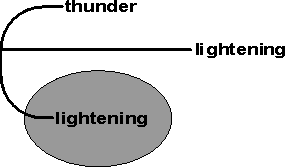Figure 4:  Result of erasing a double negation in Figure 3

Finally, the subgraph "—thunder" in the unshaded area may be erased. to produce Figure 5, which may be read as the contradictory sentence "There is lightening which is not lightening."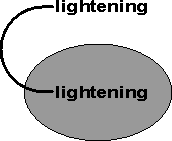Figure 5:  Result of erasure from Figure 4

Two more steps would reduce Figure 5 to Figure 6, which is the negation of empty graph. Since the empty graph is always true, its negation must always be false. To derive Figure 6 from Figure 5, use deiteration to erase the subgraph "—lightening" inside the negation. Then by the first permission, erase the subgraph "—lightening" outside the negation.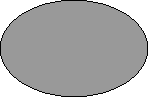Figure 6:  A universally false graph that negates the axiom

Figure 6 could also be derived from Figure 1 in just two steps. The first step is to observe that the entire graph on the left of Figure 1 is nested inside the negation on the right of Figure 1. By the rule of deiteration (permission 2), the nested graph can be erased. Then by the rule of erasure (permission 1), the graph on the left can be erased. The result is Figure 6. This universally false graph is equivalent to the empty clause, which is the universally false graph in resolution theorem provers (Robinson 1965).

To illustrate the use of Peirce's rules to prove a more complex theorem, consider Leibniz's Praeclarum Theorema (splendid theorem):

((pr) ∧ (qs)) ⊃ ((pq) ⊃ (rs)).

This formula, which has four implications, may be read "If p implies r and q implies s, then p and q implies r and s." Each of the four implications maps to a pair of a shaded and an unshaded area in Figure 7.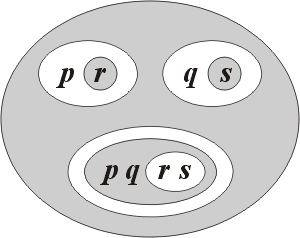Figure 7:  Leibniz's Praeclarum Theorema

Figure 8 presents a proof of Leibniz's theorem by means of Peirce's rules. Starting with an empty sheet of assertion, which is Peirce's only axiom, the proof takes seven steps. Each step is labeled with a number that indicates one of Peirce's three permissions and the letter e for an erasure or i for an insertion. The concluding graph is Figure 7.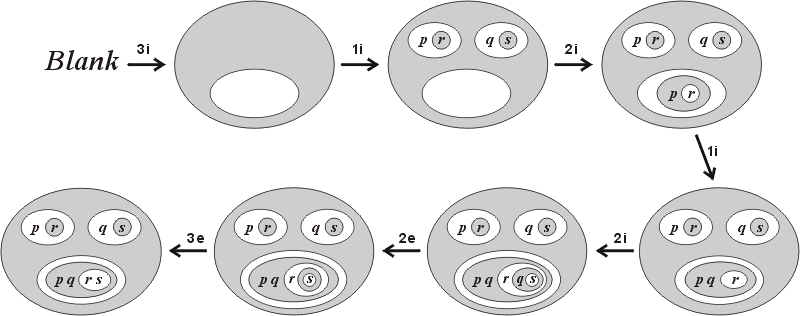Figure 8:  A proof of Leibniz's Praeclarum Theorema

The first step in Figure 8 is an application of Rule 3i to insert a double negation around the empty graph — i.e., creating a vacant ring-shaped area on a blank sheet of assertion. That operation must be the first step in the proof of any theorem, since no other rule can be applied to an emtpy sheet. The second step in proving a theorem would insert some hypothesis into the shaded area by Rule 1i. In this case, an instance of the graph corresponding to (pr)∧(qs) is inserted. Most proofs from an empty sheet would begin with an application of the rule 3i followed by 1i. The remainder of the proof would iterate subgraphs from the shaded area into the inner unshaded area by Rule 2i and apply more rules to transform the result into the desired conclusion.

In this case, the third step in Figure 8 is an application of Rule 2i to iterate a copy of the graph for pr. (An exactly equivalent proof would be obtained by iterating a copy of the graph for qs.) The fourth step applies Rule 1i to insert a copy of q into the shaded area nested three levels deep.

After only four steps, the graph looks almost like the desired conclusion, except for a missing copy of s inside the innermost area. Since that area is unshaded, it is not permissible to insert s directly by Rule 1i. Instead, Rule 2i is used to iterate a copy of the graph for qs. The next step applies Rule 2e to deiterate the unwanted copy of q in the shaded area. Finally, Rule 3e erases the vacant shaded area to derive the conclusion, QED.

In the Principia Mathematica, which Whitehead and Russell (1910) published 13 years after Peirce discovered his rules, the proof of the Praeclarum Theorema required a total of 43 steps, starting from five nonobvious axioms. One of those axioms was redundant, but the proof of its redundancy was not discovered by the authors or by any of their readers for another 16 years. All that work could have been saved if Whitehead and Russell had read Peirce's writings on existential graphs. Unfortunately, the British journal Mind is one of several that had rejected Peirce's article of 1897. That article, which was finally published in 1906 and which appears in Peirce's Collected Papers, was ignored by the overwhelming majority of 20th-century logicians.

[The remainder of this paper is incomplete.]Last Modified: